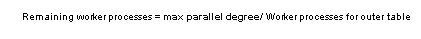# Computing the degree of parallelism for nested-loop joins

To determine the degree of parallelism for a join between any two tables (and to disqualify parallel access methods that would require too many worker processes), the optimizer applies the following rules:

1. The optimizer determines possible access methods and degrees of parallelism for the outer table of the join. This process is the same as for single-table queries.

2. For each access method determined in step 1, the optimizer calculates the remaining number of worker processes that are available for the inner table of the join. The following formula determines this number:3. The optimizer uses the remaining number of worker processes as an upper limit to determine possible access methods and degrees of parallelism for the inner table of the join.

The optimizer repeats this process for all possible join orders and access methods and applies the cost function for joins to each combination. The optimizer selects the least costly combination of join orders and access methods, and the final combination drives the degree of parallelism for the join query as a whole.

See “Nested-loop joins” for examples of this process.